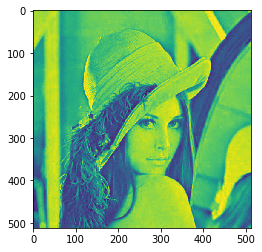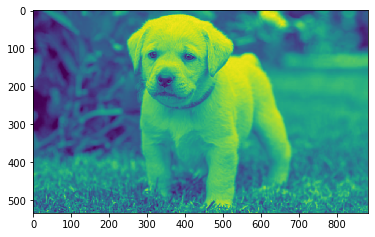Related Articles

# Mahotas – Image Ellipse Axes

• Last Updated : 26 Apr, 2021

In this article we will see how we can get the image ellipse axes in mahotas. Ellipse exes are parameters of the image ellipse, parameters of the constant intensity ellipse with the same mass and second-order moments as the original image.
For this tutorial we will use ‘lena’ image, below is the command to load the lena image

`mahotas.demos.load('lena')`

Below is the lena imageIn order to do this we will use mahotas.features.ellipse_axes method
Syntax : mahotas.features.ellipse_axes(img)
Argument : It takes image object as argument
Return : It returns two float values

Note : Input image should be filtered or should be loaded as grey
In order to filter the image we will take the image object which is numpy.ndarray and filter it with the help of indexing, below is the command to do this

`image = image[:, :, 0]`

Below is the implementation

## Python3

 `# importing required libraries``import` `mahotas``import` `mahotas.demos``from` `pylab ``import` `gray, imshow, show``import` `numpy as np``import` `matplotlib.pyplot as plt``  ` `# loading image``img ``=` `mahotas.demos.load(``'lena'``)``  ` `# filtering image``img ``=` `img.``max``(``2``)` `print``(``"Image"``)``  ` `# showing image``imshow(img)``show()` `# computing Parameters of the ‘image ellipse’``semimajor, semiminor ``=` `mahotas.features.ellipse_axes(img)`` `  `# showing value``print``(``"Semi Major Exes : "` `+` `str``(semimajor))``print``(``"Semi Minor Exes : "` `+` `str``(semiminor))`

Output :

`Image````Semi Major Exes : 295.60277400592844
Semi Minor Exes : 295.60277400592844```

Another example

## Python3

 `# importing required libraries``import` `mahotas``import` `numpy as np``from` `pylab ``import` `gray, imshow, show``import` `os``import` `matplotlib.pyplot as plt`` ` `# loading image``img ``=` `mahotas.imread(``'dog_image.png'``)`  `# fltering image``img ``=` `img[:, :, ``0``]``  ` `print``(``"Image"``)``  ` `# showing image``imshow(img)``show()``# computing Parameters of the ‘image ellipse’``semimajor, semiminor ``=` `mahotas.features.ellipse_axes(img)`` `  `# showing value``print``(``"Semi Major Exes : "` `+` `str``(semimajor))``print``(``"Semi Minor Exes : "` `+` `str``(semiminor))`

Output :

`Image````Semi Major Exes : 508.79612573247636
Semi Minor Exes : 308.5809619544451```

Attention geek! Strengthen your foundations with the Python Programming Foundation Course and learn the basics.

To begin with, your interview preparations Enhance your Data Structures concepts with the Python DS Course. And to begin with your Machine Learning Journey, join the Machine Learning – Basic Level Course

My Personal Notes arrow_drop_up